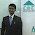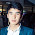## Image Processing : Edge Detection of Image Using MATLAB

There are many operators in MATLAB for finding images
1. Sobels Operator
2. Roberts Operator
3. Prewitts Operator
4. Laplacian of Gaussian Method
5. Zero-Cross Method, etc..

To use them in MATLAB there is a function called as edge(I).

Syntax :

```BW = edge(I)

BW = edge(I,'sobel')```

`BW = edge(I,'prewitt')`
` `
`BW = edge(I,'roberts') etc.`
```
```
`Description : `

BW = edge(I) takes a grayscale or a binary image I as its input, and returns a binary image BW of the same size as I, with 1's where the function finds edges in I and 0's elsewhere.

By default, edge uses the Sobel method to detect edges but the following provides a complete list of all the edge-finding methods supported by this function:
• The Sobel method finds edges using the Sobel approximation to the derivative. It returns edges at those points where the gradient of I is maximum.
• The Prewitt method finds edges using the Prewitt approximation to the derivative. It returns edges at those points where the gradient of I is maximum.
• The Roberts method finds edges using the Roberts approximation to the derivative. It returns edges at those points where the gradient of I is maximum.
• The Laplacian of Gaussian method finds edges by looking for zero crossings after filtering I with a Laplacian of Gaussian filter.
• The zero-cross method finds edges by looking for zero crossings after filtering I with a filter you specify.
• The Canny method finds edges by looking for local maxima of the gradient of I. The gradient is calculated using the derivative of a Gaussian filter. The method uses two thresholds, to detect strong and weak edges, and includes the weak edges in the output only if they are connected to strong edges. This method is therefore less likely than the others to be fooled by noise, and more likely to detect true weak edges.
The parameters you can supply differ depending on the method you specify. If you do not specify a method, edge uses the Sobel method.

Example :

Find the edges of an image using the Prewitt , Sobel and Roberts methods.
` `

 ```i = imread('far.jpg'); I = rgb2gray(i); BW1 = edge(I,'prewitt'); BW2= edge(I,'sobel'); BW3= edge(I,'roberts'); subplot (2,2,1); imshow(I); title('original'); subplot(2,2,2); imshow(BW1); title('Prewitt'); subplot(2,2,3); imshow(BW2); title('Sobel'); subplot(2,2,4); imshow(BW3); title('Roberts'); ``` ` `

SHARE THIS POST:

#### 13 comments:

1.The description is quite useful.

2.Thanks Aaron..

3.NICE !!!!

4.where can i find edge code definition please?

5.but when we convert RGB to gray there is lost of some infomation. About 90% information in RGB image is same as that of gray scale image but remaining 10% is not same.my question is that what can I do for getting this 10% info(i.e brightness,hue and saturation).

6.@Matlabhelp While using rgb2gray in MATLAB we have a percentage error of 0.2295 where if we use BRAUNN Method then we have .1195. that is 10%. But according to some the conversion would not have any effect on the geometric accuracy of
your image. All you are loosing is the information regarding the
colour of each pixel.
Recovery of that i have no idea.

7.In my project there is one step:-RGB image edge detection using component gradient operators.
This involves the following steps
1> The R,G and B component is computed.
2> The component is separately processed using the way of gradient operator.
3>The RGB color image is given through the processed separate components

8.@Matlabhelp What you have described in the 1st step is what is normally done to change an image in to gray scale, i.e. RGB component intensity is computed. Matlab func. rgb2gray also computes r,g, n b components and applies this formula : 0.2989 * R + 0.5870 * G + 0.1140 * B .

what you are doing is also right you have a gradient operator to use with it.

9.You spelled Sobel wrong

10.as i am new in matlab ,please tell me how to run this command.

11.i want program on steganography

12.i want program on steganography

13.Very useful example for methods